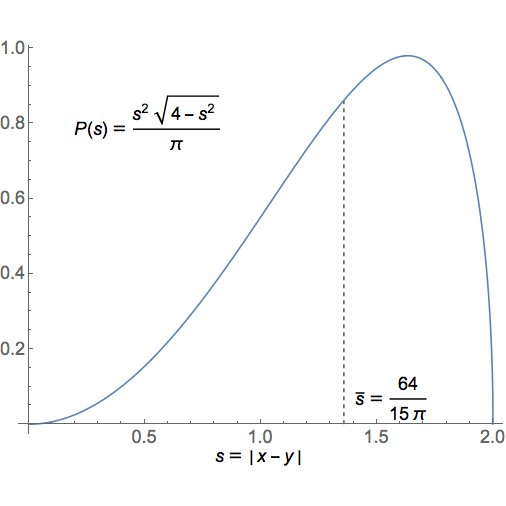Recently [Greg Egan and I were talking about quaternions](https://twitter.com/gregeganSF/status/1015178113347608576), and I asked him to compute the mean distance between two randomly chosen unit quaternions.

You don't need to know anything about quaternions to do this. Another way to phrase the question is: what's the average distance between two randomly chosen points of the unit sphere in \$$\mathbb{R}^4\$$?

Yet another way to put it is this: if you sit at the north pole of this sphere, and randomly pick another point on the sphere, what is the expected value of its distance from you?"

(Here and in all that follows, distances are measured using straight lines in \$$\mathbb{R}^n\$$, not using geodesics on the sphere. There is a unique rotation-invariant probability measure on a sphere of any dimension, and that's what we use to randomly choose a point on a sphere.)

Greg computed the answer, which is \$$64/15\pi \approx 1.35812218105\$$. But he went further and computed the whole probability distribution:I tried to figure out why the answer looked like this. I noticed, that if if you take a unit sphere in any dimension, and you sit at the north pole, the points on the equator are at distance \$$\sqrt{2} \approx 1.41421356\$$. This is pretty close to \$$1.35812218105\$$.

I wrote:

> Neat! I'm kind of surprised the mode doesn't occur at $\sqrt(2)$, because if you're at the north pole it'll seem like "most" points are at the equator. Maybe I'm making an elementary mistake, confusing parametrization by angle and by distance.

That was indeed the problem.

But as you go to higher dimensions, points near the equator should become more and more probable (you can see this using a little calculation). So, I predicted that if we repeat this calculation for spheres of different dimensions, the probability distribution should spike more and more strongly near \$$\sqrt{2}\$$.